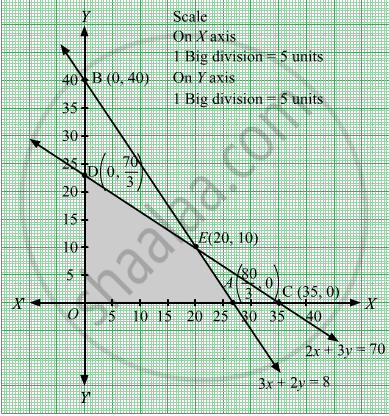# Maximize Z = 15x + 10y Subject to 3 X + 2 Y ≤ 80 2 X + 3 Y ≤ 70 X , Y ≥ 0 - Mathematics

Sum

Maximize Z = 15x + 10y
Subject to

$3x + 2y \leq 80$
$2x + 3y \leq 70$
$x, y \geq 0$

#### Solution

First, we will convert the given inequations into equations, we obtain the following equations:
3x + 2y = 80, 2x + 3y = 70, x = 0 and y = 0
Region represented by 3x + 2y ≤ 80 :
The line 3x + 2y = 80 meets the coordinate axes at $A\left( \frac{80}{3}, 0 \right)$ and  $B\left( 0, 40 \right)$respectively. By joining these points we obtain the line 3x + 2y = 80.
Clearly (0,0) satisfies the inequation 3x + 2y ≤ 80 . So,the region containing the origin represents the solution set of the inequation 3x + 2y ≤ 80 .
Region represented by 2x + 3y ≤ 70:
The line 2x + 3y = 70 meets the coordinate axes at $C\left( 35, 0 \right)$ and  $D\left( 0, \frac{70}{3} \right)$ respectively. By joining these points we obtain the line 2x + 3y ≤ 70.
Clearly (0,0) satisfies the inequation 2x + 3y ≤ 70. So,the region containing the origin represents the solution set of the inequation 2x + 3y ≤ 70.
Region represented by x ≥ 0 and y ≥ 0:
Since, every point in the first quadrant satisfies these inequations. So, the first quadrant is the region represented by the inequations x ≥ 0 and ≥ 0.
The feasible region determined by the system of constraints, 3x + 2y ≤ 80, 2x + 3y ≤ 70, x ≥ 0, and y ≥ 0 are as follows.The corner points of the feasible region are O(0, 0), $A\left( \frac{80}{3}, 0 \right)$ $E\left( 20, 10 \right)$ and $D\left( 0, \frac{70}{3} \right)$

The values of Z at these corner points are as follows.

 Corner point Z = 15x + 10y O(0, 0) 15 × 0 + 10 × 0 = 0 $A\left( \frac{80}{3}, 0 \right)$ 15 × $\frac{80}{3}$+ 10 × 0 = 400 $E\left( 20, 10 \right)$ 15 × 20 + 10 × 10 = 400 $D\left( 0, \frac{70}{3} \right)$ 15 × 0 + 10 × $\frac{70}{3}$ =$\frac{700}{3}$

We see that the maximum value of the objective function Z is 400 which is at $A\left( \frac{80}{3}, 0 \right)$ and $E\left( 20, 10 \right)$ Thus, the optimal value of Z is 400.

Concept: Graphical Method of Solving Linear Programming Problems
Is there an error in this question or solution?

#### APPEARS IN

RD Sharma Class 12 Maths
Chapter 30 Linear programming
Exercise 30.2 | Q 6 | Page 32